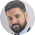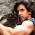# Playing with Percentages - 7 Techniques to Learn

The old idiom goes, ‘there are no shortcuts to success’; we have probably heard this one at least a hundred times in our life already.

But times are changing and in our present situation, where we are students and candidates of competitive exams…shortcuts are the only way to success!

Think Math – think shortcuts; whether the shortcuts are your own methods or taught by someone, or learnt from a book – they need to be remembered and inculcated into your normal ‘mathematical’ thought process.
You should always solve sums with minimal calculations, and if you have got the ‘mental calculations’ power – you’re really one lucky person! Make use of your abilities and answer all 50 questions!

That done, we begin with our topic for the day Percentages

I’m starting with this topic as percentages is required in both the normal math questions as well data interpretation.

(Has anyone noticed the SBI Associates PO official notice says its 50 questions of DI& DA?!)

### Technique 1

If out of the total apples 60% are bad, i.e., 100% - 60% = 40% is good.
So, when we are asked the number of good apples, simply do the mental calc (short

form of calculation!) and get the figure of 40%, the multiply with the total number of

apples say 600, which is :

40 x 600 / 100 = 240 apples

### Technique 2

You already know that the ‘00s in 600 and ‘00s in 100 get cancelled, then why waste time writing down the entire figure?

Simply multiply 40 x 6 = 240!

### Technique 3

Also, 40% can be written as 0.4 or simply .4 – how?
40/100 is 0.4!

So you can multiply 600 with 0.4 and put the decimal point to get 240!

When I say multiply with 0.4, you should actually multiply with 4, (i.e. 600 x 4), which
gives us 2400 and then put a decimal. Gives you 240.

### Technique 4

In DI

Where the total number of students is given as 1950, and you are asked to find the total of Computer and Bio students, what do you do?

You add up their respective percentages and then find the total figure.

28 + 26 = 54%

54/100 * 1950 = 1053 (I’m showing the complete calculations, but you are to always use the shortcuts!)

If they ask the difference between the number of students in Chemistry and Commerce…then 21 – 15 = 6% and 1950 x 6% =117 students.

If they ask the difference between the number of students studying computer and commerce and chemistry and arts…

We add up the % figures of computer and commerce = 43%,
and of chemistry and arts which is = 31%, and then get their difference = 43-31= 12 %,
thus, 12%  of 1950 = 234 students.

Please Note: This method can only work when only percentages are given; where ratios are also given the method of calculation will be different. We’ll discuss that when doing Ratio’s chapter.

### Technique 5

Price of commodity! Bank PO’s favorite question!

The short cut in these questions is the formulae which is printed in every quantitative aptitude book!

Suppose if the Price of Oranges increases by 5%, then the reduction required in the consumption so as to maintain the original expenditure will be = 5/(100+5) x 100 =

4.76%. Here, reduction can only be calculated in % form as absolute figure is not given, i.e., number of oranges is not given. [ formula is R/(100+R) * 100]

Similarly, if the price of oranges decreases by 5%, then the % increase in the consumption so as to maintain the original expenditure = 5/(100-95) x 100 = 5.26% [formula is R/(100+R) *100)

If you are having a problem remembering the formula, then do it this way – the

numerator will always be the percentage number, in our case ‘5’.

If the price is increasing by ‘5’%, then in the denominator we add 5 to 100, i.e., 105.
If the price is decreasing by ‘5’%, then in the denominator we subtract 5 from 100, i.e., 95.

### Technique 6

% and Population! Another one of the PO googlies!

Here, just remember one thing…population increase is exactly the same as compound

interest! Use the same concept here too…

If the population of a town is, say 20,000 people as of today, and if population

increases at 6% p.a., then what will be the population after 3 year?

Do you see the similarity between this question and a compound interest question?

Except for the semi-annually or quarterly compounding part, every other information

can be seen in a similar fashion and that is why their formulae are same too!

Population after 3 years = 20,000 x (1 + 6/100)3 = 23820 approx
Formula being – Population x (1 +R/100)n
where, n is the number of years.

If population is decreasing, we simply remove the ‘+’ sign and put a ‘–’ instead, i.e.,

Population x (1 – R/100)n

Population increase or decrease in future is called calculation of a ‘future value’ and

hence this formulae, where we multiply with the present population holds true.

But when calculating a past value, we will need to divide the present population by the

% increase/decrease for the required number of years.

Thus our formula is, P/ (1+ R/100)n or P/ (1- R/100)n
or increase or decrease in population respectively!

### Technique 7

The above mentioned formulae and concepts hold true for depreciation sums as well. Depreciation means, the decrease in an assets value due to wear and tear or usage.

When you purchase a mobile, Nokia Lumia or Samsung Galaxy, and suppose it costs you Rs.15,000 today. You use it for a year, and then you want to sell it, how much will you ask for? Rs.15000? How much will you get? Rs.15000? Surely not!

Why not? The value goes down from 15000, but why?

Because you and the buyer both know that once any asset has been used, it most probably will have some defects (scratched screen, weak battery, and phone getting way too warm).

So, why will you pay the same amount for a defective piece, which you pay for the new phone! But what is the value of the old phone? How to get the proper value of the old phone?

This is where the concept and formula of depreciation (which is similar to the population formulae) come to help!

Like I said before, in math, shortcuts are important and developing your own methods to solve problems quickly is even more important.

Daily and rigorous practice only will get you through the 50 question.

Aim higher and you will definitely achieve it.

Till then keep the feedbacks coming in.#### What's trending in BankExamsToday

Smart Prep Kit for Banking Exams by Ramandeep Singh - Download here1.hello sir.. is there any chance for 41.2 reserve list student in PO3??

2.copied from M.tyra book...

3.Great sir !!!

4.copy cat raman pata nai phir se kya copy kar raha hoga .. mystry

1.go find it out !! y r u wasting ur tym in commenting ovr here ?? n i dont understand if he is copying n elaborating sum points frm sum source then wats wrng in dat .. he cant develop his own formulae fr maths now ... n stop criticizing plzz

2.Hi there,

I am not a scientist. Neither this is a carbon copy of any book. If it is, then show me the source.

Prize - Rs 10,000

5.thank you so much sir.this is really helpful... kindly give us some ratio and proportion tricks.

6.7.agar copy kar bhi raha h toh atleast unhe logon tak pahuncha k acha kaam hi kar rha h...tum logo ne kya kiya aaj tak...sala koi acha kaam kare toh uski push back karo bas..
u r doing grt job Raman Sir. Keep it up

1.well said dear :)

2.Hey it's not copied.

Many times there is some common content in all websites. If RBI is declaring results and everybody will shave to share the news. I never copy ideas or content

8.Sir SBI associates PO ki expected exam date kya ho sakti h???

9.Wish you and ur family happy diwal.

1.Happy Diwali

10.11.